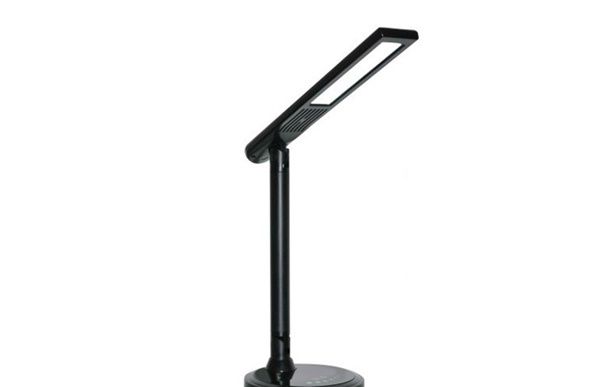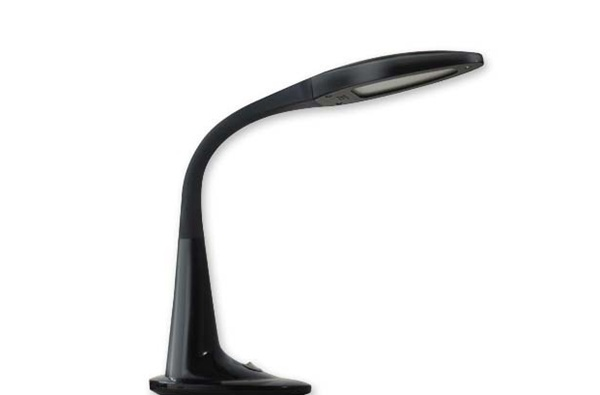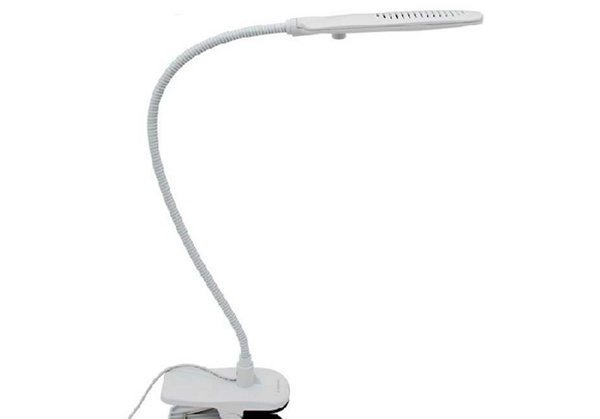|

# LED护眼灯好吗 LED护眼灯选购方法

为了孩子能够有一个良好的学习环境，家长在准备书桌椅的同时，当然也少不了一款合适的护眼灯产品，那哪种护眼灯比较好呢？LED护眼灯好吗？【LED护眼灯好吗】

led护眼灯就是以led(Light Emitting Diode)即发光二极管为光源的节能护眼灯，led是一种固态的半导体器件，它可以直接把电转化为光。led护眼灯把低频闪提高至高频闪，将灯管的亮灭闪烁加快到每秒几千次甚至几万次，闪烁的速度超过了人眼的神经反应速度，眼睛就感觉不到闪烁，因此对眼睛起到一定的保护作用。

1：环保型灯具

传统的日光灯中含有大量的水银蒸汽，如果破碎水银蒸汽则会挥发到大气中。但LED日光灯则根本不使用水银，且LED产品也不含铅，对环境起到保护作用。LED日光灯公认为二十一世纪的绿色照明。

2：发热少，电能利用率高

传统灯具在点亮后会产生大量的热能，降低了电能的利用率，而LED灯具在点亮的过程中产生的热量很少（小功率芯片做的LED灯产生的热量更小，人手可以安全触摸而不感到烫手）电能到光能的转换率接近100%，不会造成能源的浪费。而且对文件，衣物会有保护作用，不会出现像普通的灯光下所出现的文件衣物的褪色现象。

3：没有噪音

LED灯具不会产生噪音，对于使用精密电子仪器的场合为上佳之选。适合于图书馆，办公室之类的场合。4：保护眼睛

传统的日光灯使用的是交流电，所以每秒钟会产生100－120次的频闪，频闪效应会引发视觉疲劳、偏头痛，对眼睛造成疲劳和伤害，在我国80年代以后，T8直管型（电感式）日光灯，普遍应用于家庭、学校、图书馆等，成长中的中小学生受害极大，视力下降明显，近视眼显着增多。LED灯具是把交流电直接转换为直流电，没有频闪不会产生闪烁现象，保护眼睛。

5：无蚊虫烦恼

LED灯具不会产生紫外线，因此不会象传统的灯具那样，有很多蚊虫围绕在灯源旁。室内会变得更加干净卫生整洁。

6：宽电压工作，在85V－265V 电压之间均可正常工作

传统的日光灯是通过整流器释放的高电压来点亮的，当电压降低时则无法点亮。而LED灯具在一定范围的电压之内都能点亮，还能调整光亮度.

7：省电寿命长

LED日光灯的耗电量是传统日光灯的三分之一以下，寿命也是传统日光灯的10倍以上，可以长期使用而无需更换，减少人工费用。更适合于难于更换的场合。

8：坚固牢靠

LED灯体本身使用的是环氧树脂而并非传统的玻璃，更坚固牢靠，易于长途运输，即使砸在地板上LED也不会轻易损坏，可以放心地使用，也避免了普通日光在长途运输中出现的易碎的现象。【LED护眼灯选购方法】

1、外观质量

理想的阅读光源应具备接近自然光、无频闪、亮度可调光4至6档及光照均匀等特点。另外，产品准产证、质量监检号等各种证件也要齐全。

2、色温值

灯管发光的色温值决定了灯光的柔和度，太高和太低都不太好，4000K至5200K比较适合。其中4000K至4500K的护眼灯光线稍微偏红，更加柔和，适合小学生使用;而4500K至5200K的色温值适合中学生及其以上年龄的顾客使用。

3、售后服务

在新光源技术越来越先进的今天，led护眼灯的使用、保养方法尤为重要，因此购买时优先选购知名品牌和100元以上的产品。在购买点，厂商人员会当场演示、讲解，直到消费者掌握并理解使用方式，而且知名品牌有充足的备件，如灯管，以保证不时之需。【LED护眼灯品牌推荐】

LED护眼灯哪个好-欧司朗

欧司朗，中国灯具十大品牌之一，也是中国led灯十大品牌。灯具行业的知名品牌。要是对护眼灯哪个好不甚了解。就不妨去看一看欧司朗品牌的护眼灯吧！世界知名灯具的制造厂商，也是最早的灯具照明企业，欧司朗从业多年，在研究光源、制造灯具方面拥有娴熟的技术，强悍的研发团队，欧司朗品牌的护眼灯，价格实惠、外观时尚靓丽，深受很多消费者的青睐！护眼灯哪个好，欧司朗值得推荐！

LED护眼灯哪个好-好视力

好视力护眼灯，就像这个品牌的名字一样，专门致力于健康光源的研发和制造。护眼灯哪个好，好视力护眼灯，灯珠分布均匀，无阴影、无盲区，光线效果非常好，还可以帮助孩子学习，减少视觉疲劳，让孩子在好视力护眼灯之下，学习、看书、写字不疲劳，让眼睛更舒适。好视力护眼灯，保护孩子的好视力。如果你还是无法确定护眼灯哪个好，不妨多看一看好视力护眼灯，真的很不错！LED护眼灯哪个好-欧普

对于护眼灯的选择，护眼灯哪个好，就要从品牌、质量、性能、服务以及价格等几个方面去选择，这五个方面包括了护眼灯选择的各个方面，也可以看出护眼灯品牌的实力。而欧普在这个几个方面，虽然并不是做得十全十美，但是起码的十全九美确实达到了的。欧普也是中国灯具十大品牌之一，在研发创新护眼灯方面也有十足的实力。护眼灯哪个好，欧普护眼灯也是值得信赖的！

`声明：本文由入驻焦点开放平台的作者撰写，除焦点官方账号外，观点仅代表作者本人，不代表焦点立场错误信息举报电话： 400-099-0099，邮箱：jubao@vip.sohu.com，或点此进行意见反馈，或点此进行举报投诉。`A B C D E F G H J K L M N P Q R S T W X Y Z
A - B - C - D - E
• A
• 鞍山
• 安庆
• 安阳
• 安顺
• 安康
• 澳门
• B
• 北京
• 保定
• 包头
• 巴彦淖尔
• 本溪
• 蚌埠
• 亳州
• 滨州
• 北海
• 百色
• 巴中
• 毕节
• 保山
• 宝鸡
• 白银
• 巴州
• C
• 承德
• 沧州
• 长治
• 赤峰
• 朝阳
• 长春
• 常州
• 滁州
• 池州
• 长沙
• 常德
• 郴州
• 潮州
• 崇左
• 重庆
• 成都
• 楚雄
• 昌都
• 慈溪
• 常熟
• D
• 大同
• 大连
• 丹东
• 大庆
• 东营
• 德州
• 东莞
• 德阳
• 达州
• 大理
• 德宏
• 定西
• 儋州
• 东平
• E
• 鄂尔多斯
• 鄂州
• 恩施
F - G - H - I - J
• F
• 抚顺
• 阜新
• 阜阳
• 福州
• 抚州
• 佛山
• 防城港
• G
• 赣州
• 广州
• 桂林
• 贵港
• 广元
• 广安
• 贵阳
• 固原
• H
• 邯郸
• 衡水
• 呼和浩特
• 呼伦贝尔
• 葫芦岛
• 哈尔滨
• 黑河
• 淮安
• 杭州
• 湖州
• 合肥
• 淮南
• 淮北
• 黄山
• 菏泽
• 鹤壁
• 黄石
• 黄冈
• 衡阳
• 怀化
• 惠州
• 河源
• 贺州
• 河池
• 海口
• 红河
• 汉中
• 海东
• 怀来
• I
• J
• 晋中
• 锦州
• 吉林
• 鸡西
• 佳木斯
• 嘉兴
• 金华
• 景德镇
• 九江
• 吉安
• 济南
• 济宁
• 焦作
• 荆门
• 荆州
• 江门
• 揭阳
• 金昌
• 酒泉
• 嘉峪关
K - L - M - N - P
• K
• 开封
• 昆明
• 昆山
• L
• 廊坊
• 临汾
• 辽阳
• 连云港
• 丽水
• 六安
• 龙岩
• 莱芜
• 临沂
• 聊城
• 洛阳
• 漯河
• 娄底
• 柳州
• 来宾
• 泸州
• 乐山
• 六盘水
• 丽江
• 临沧
• 拉萨
• 林芝
• 兰州
• 陇南
• M
• 牡丹江
• 马鞍山
• 茂名
• 梅州
• 绵阳
• 眉山
• N
• 南京
• 南通
• 宁波
• 南平
• 宁德
• 南昌
• 南阳
• 南宁
• 内江
• 南充
• P
• 盘锦
• 莆田
• 平顶山
• 濮阳
• 攀枝花
• 普洱
• 平凉
Q - R - S - T - W
• Q
• 秦皇岛
• 齐齐哈尔
• 衢州
• 泉州
• 青岛
• 清远
• 钦州
• 黔南
• 曲靖
• 庆阳
• R
• 日照
• 日喀则
• S
• 石家庄
• 沈阳
• 双鸭山
• 绥化
• 上海
• 苏州
• 宿迁
• 绍兴
• 宿州
• 三明
• 上饶
• 三门峡
• 商丘
• 十堰
• 随州
• 邵阳
• 韶关
• 深圳
• 汕头
• 汕尾
• 三亚
• 三沙
• 遂宁
• 山南
• 商洛
• 石嘴山
• T
• 天津
• 唐山
• 太原
• 通辽
• 铁岭
• 泰州
• 台州
• 铜陵
• 泰安
• 铜仁
• 铜川
• 天水
• 天门
• W
• 乌海
• 乌兰察布
• 无锡
• 温州
• 芜湖
• 潍坊
• 威海
• 武汉
• 梧州
• 渭南
• 武威
• 吴忠
• 乌鲁木齐
X - Y - Z
• X
• 邢台
• 徐州
• 宣城
• 厦门
• 新乡
• 许昌
• 信阳
• 襄阳
• 孝感
• 咸宁
• 湘潭
• 湘西
• 西双版纳
• 西安
• 咸阳
• 西宁
• 仙桃
• 西昌
• Y
• 运城
• 营口
• 盐城
• 扬州
• 鹰潭
• 宜春
• 烟台
• 宜昌
• 岳阳
• 益阳
• 永州
• 阳江
• 云浮
• 玉林
• 宜宾
• 雅安
• 玉溪
• 延安
• 榆林
• 银川
• Z
• 张家口
• 镇江
• 舟山
• 漳州
• 淄博
• 枣庄
• 郑州
• 周口
• 驻马店
• 株洲
• 张家界
• 珠海
• 湛江
• 肇庆
• 中山
• 自贡
• 资阳
• 遵义
• 昭通
• 张掖
• 中卫

1室1厅1厨1卫1阳台

1
2
3
4
5

0
1
2

1

1

0
1
2
3报名成功，资料已提交审核A B C D E F G H J K L M N P Q R S T W X Y Z
A - B - C - D - E
• A
• 鞍山
• 安庆
• 安阳
• 安顺
• 安康
• 澳门
• B
• 北京
• 保定
• 包头
• 巴彦淖尔
• 本溪
• 蚌埠
• 亳州
• 滨州
• 北海
• 百色
• 巴中
• 毕节
• 保山
• 宝鸡
• 白银
• 巴州
• C
• 承德
• 沧州
• 长治
• 赤峰
• 朝阳
• 长春
• 常州
• 滁州
• 池州
• 长沙
• 常德
• 郴州
• 潮州
• 崇左
• 重庆
• 成都
• 楚雄
• 昌都
• 慈溪
• 常熟
• D
• 大同
• 大连
• 丹东
• 大庆
• 东营
• 德州
• 东莞
• 德阳
• 达州
• 大理
• 德宏
• 定西
• 儋州
• 东平
• E
• 鄂尔多斯
• 鄂州
• 恩施
F - G - H - I - J
• F
• 抚顺
• 阜新
• 阜阳
• 福州
• 抚州
• 佛山
• 防城港
• G
• 赣州
• 广州
• 桂林
• 贵港
• 广元
• 广安
• 贵阳
• 固原
• H
• 邯郸
• 衡水
• 呼和浩特
• 呼伦贝尔
• 葫芦岛
• 哈尔滨
• 黑河
• 淮安
• 杭州
• 湖州
• 合肥
• 淮南
• 淮北
• 黄山
• 菏泽
• 鹤壁
• 黄石
• 黄冈
• 衡阳
• 怀化
• 惠州
• 河源
• 贺州
• 河池
• 海口
• 红河
• 汉中
• 海东
• 怀来
• I
• J
• 晋中
• 锦州
• 吉林
• 鸡西
• 佳木斯
• 嘉兴
• 金华
• 景德镇
• 九江
• 吉安
• 济南
• 济宁
• 焦作
• 荆门
• 荆州
• 江门
• 揭阳
• 金昌
• 酒泉
• 嘉峪关
K - L - M - N - P
• K
• 开封
• 昆明
• 昆山
• L
• 廊坊
• 临汾
• 辽阳
• 连云港
• 丽水
• 六安
• 龙岩
• 莱芜
• 临沂
• 聊城
• 洛阳
• 漯河
• 娄底
• 柳州
• 来宾
• 泸州
• 乐山
• 六盘水
• 丽江
• 临沧
• 拉萨
• 林芝
• 兰州
• 陇南
• M
• 牡丹江
• 马鞍山
• 茂名
• 梅州
• 绵阳
• 眉山
• N
• 南京
• 南通
• 宁波
• 南平
• 宁德
• 南昌
• 南阳
• 南宁
• 内江
• 南充
• P
• 盘锦
• 莆田
• 平顶山
• 濮阳
• 攀枝花
• 普洱
• 平凉
Q - R - S - T - W
• Q
• 秦皇岛
• 齐齐哈尔
• 衢州
• 泉州
• 青岛
• 清远
• 钦州
• 黔南
• 曲靖
• 庆阳
• R
• 日照
• 日喀则
• S
• 石家庄
• 沈阳
• 双鸭山
• 绥化
• 上海
• 苏州
• 宿迁
• 绍兴
• 宿州
• 三明
• 上饶
• 三门峡
• 商丘
• 十堰
• 随州
• 邵阳
• 韶关
• 深圳
• 汕头
• 汕尾
• 三亚
• 三沙
• 遂宁
• 山南
• 商洛
• 石嘴山
• T
• 天津
• 唐山
• 太原
• 通辽
• 铁岭
• 泰州
• 台州
• 铜陵
• 泰安
• 铜仁
• 铜川
• 天水
• 天门
• W
• 乌海
• 乌兰察布
• 无锡
• 温州
• 芜湖
• 潍坊
• 威海
• 武汉
• 梧州
• 渭南
• 武威
• 吴忠
• 乌鲁木齐
X - Y - Z
• X
• 邢台
• 徐州
• 宣城
• 厦门
• 新乡
• 许昌
• 信阳
• 襄阳
• 孝感
• 咸宁
• 湘潭
• 湘西
• 西双版纳
• 西安
• 咸阳
• 西宁
• 仙桃
• 西昌
• Y
• 运城
• 营口
• 盐城
• 扬州
• 鹰潭
• 宜春
• 烟台
• 宜昌
• 岳阳
• 益阳
• 永州
• 阳江
• 云浮
• 玉林
• 宜宾
• 雅安
• 玉溪
• 延安
• 榆林
• 银川
• Z
• 张家口
• 镇江
• 舟山
• 漳州
• 淄博
• 枣庄
• 郑州
• 周口
• 驻马店
• 株洲
• 张家界
• 珠海
• 湛江
• 肇庆
• 中山
• 自贡
• 资阳
• 遵义
• 昭通
• 张掖
• 中卫• 手机• 分享
• 设计
免费设计
• 计算器
装修计算器
• 入驻
合作入驻
• 联系
联系我们
• 置顶
返回顶部